Question
1. An AM receiver is tuned to broadcast station at 600 kHz. Calculate the image rejection in dB, assuming that the input filter consist of one tuned circuit with a Q of 40?
2. An SSB system transmit a peak envelope power of 100 W into a 75 ohms load. The carrier signal is modulated by two tones, with frequencies 2 kHz and 3 kHz with equal magnitude and only the lower sideband is transmitted with a suppressed carrier. Determine the average power into the load?
3. In the SSB, determine the maximum suppression of the unwanted sideband if the deviation from a perfect 90 phase shift is 5”?
4. An AM transmitter has an output power of 100 W at no modulation with efficiency of 60 %. What will be the output power with 97 % modulation?
5. Given an audio power of 500 W which will modulated an RF amplifier to 50 % modulate an RF amplifier to 50 % modulation. Find the DC power input?
6. A CB transmitter supplies a total power of 120 W to an aerial. Suppose the carrier is amplitude modulated to a depth of 80 %, how much power is wasted by the carrier signal?
7. Determine the modulation index of the signal given the following parameters: Emax = 10 Vp, Em= 4 Vp.
8. A DSB-SC system must suppress the carrier by 50 dB for its original value of 10 W. To what value must the carrier be reduced?
9. A transistor has a power dissipation rating of 30 W. Assuming that the transistor is the only element that dissipates power in a circuit, calculate the power an amplifier, using this transistor, could deliver the load if it operates as Class-A wit an efficiency of 30 %?
10. An AM signal supplies 150 W of carrier power when it is modulated by a single sine wave to a depth of 70 %. Suppose the total transmitted power increases by 10 % when another sine wave simultaneously amplitude modulates the carrier, determine the modulation index of the second sine wave?

Ans 1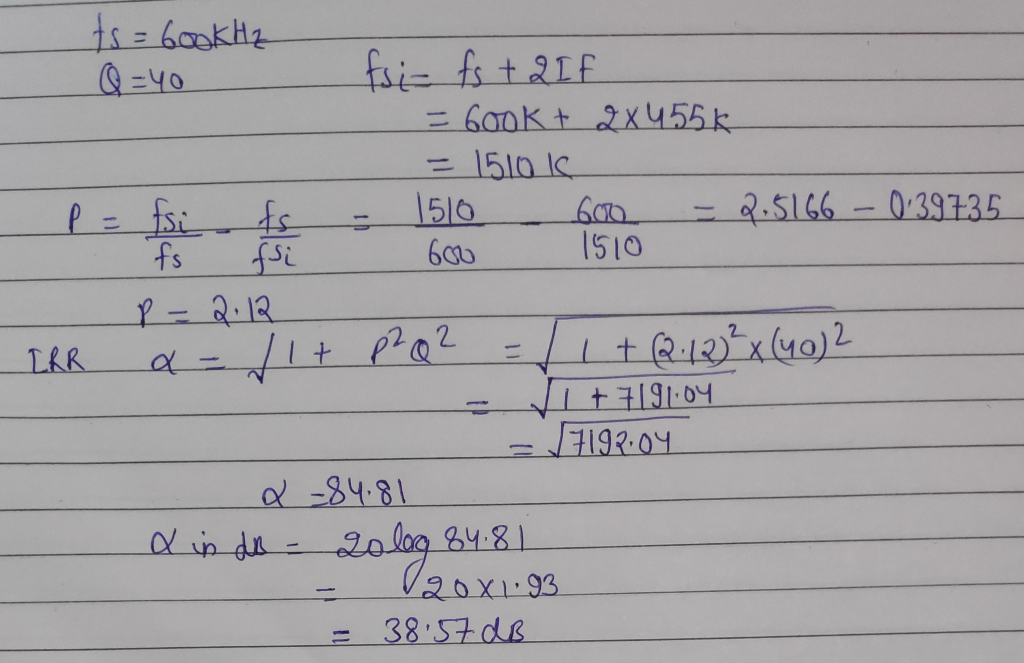#### Earn Coins

Coins can be redeemed for fabulous gifts.

Similar Homework Help Questions
• ### An AM receiver is tuned to broadcast station at 600 kHz. Calculate the image rejection in dB, assuming that the input f...

An AM receiver is tuned to broadcast station at 600 kHz. Calculate the image rejection in dB, assuming that the input filter consist of one tuned circuit with a Q of 40? An SSB system transmit a peak envelope power of 100 W into a 75 ohms load. The carrier signal is modulated by two tones, with frequencies 2 kHz and 3 kHz with equal magnitude and only the lower sideband is transmitted with a suppressed carrier. Determine the average...

• ### 71. A superheterodyne receiver tunes at the frequency range from 25 to 50 MHz. What is the IF frequency of the rece...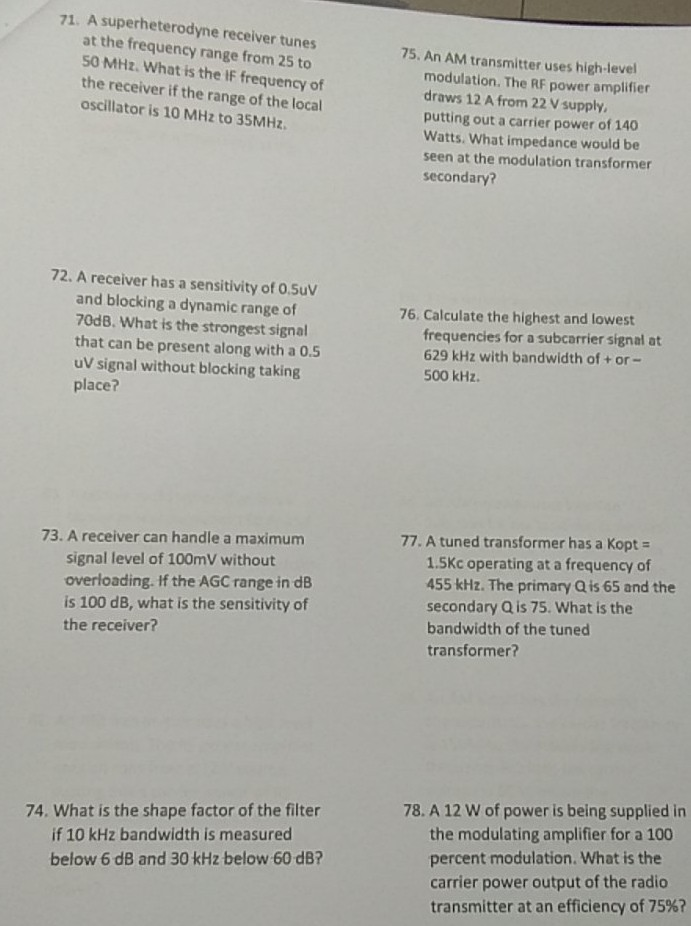71. A superheterodyne receiver tunes at the frequency range from 25 to 50 MHz. What is the IF frequency of the receiver if the range of the local oscillator is 10 MHz to 35MHz 75. An AM transmitter uses high-level modulation. The RF power amplifier draws 12 A from 22 V supply putting out a carrier power of 140 Watts. What impedance would be seen at the modulation transformer secondary? 72. A receiver has a sensitivity of 0Suv and blocking...

• ### The AM signal supplies 150w of carrier power when it is modulated by a single sine wave to a depth of 70%. Suppose the total transmitted power increases by 10% when another sine wave simultaneously amplitude modulates the carrier. Determine the modulation

The AM signal supplies 150w of carrier power when it is modulated by a single sine wave to a depth of 70%. Suppose the total transmitted power increases by 10% when another sine wave simultaneously amplitude modulates the carrier. Determine the modulation index of the second index.

• ### ()=< a) Consider the case where a modulating tone v, a cos(2ft) is used in a...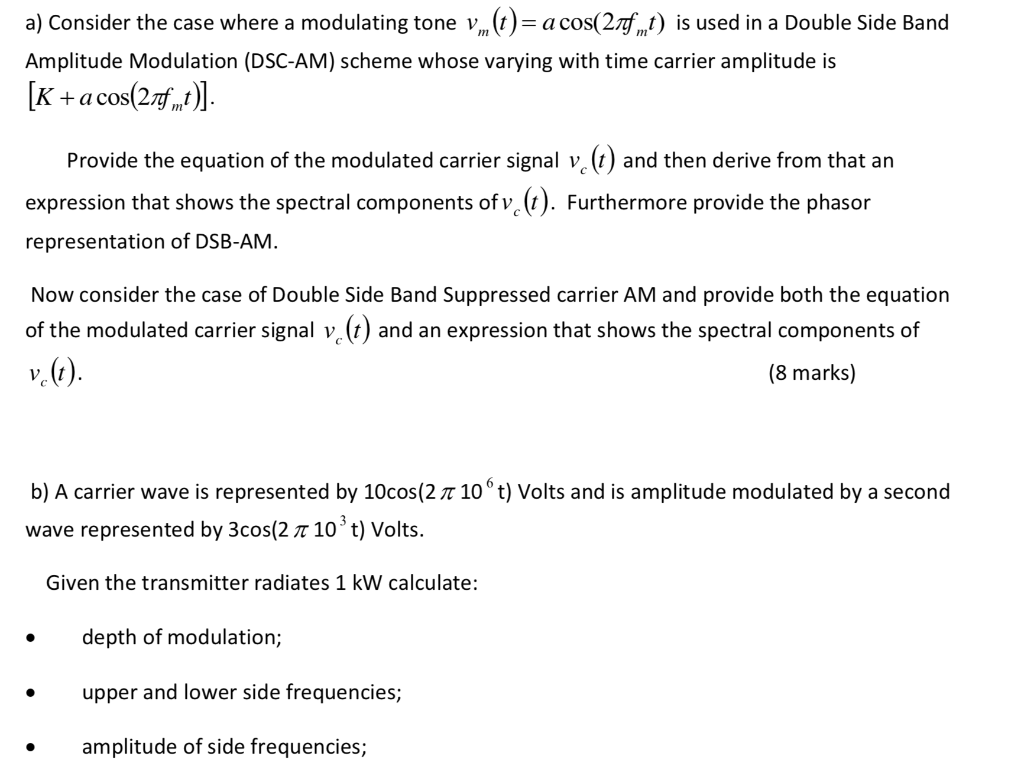()=< a) Consider the case where a modulating tone v, a cos(2ft) is used in a Double Side Band m Amplitude Modulation (DSC-AM) scheme whose varying with time carrier amplitude is [K+a cos(2ft Provide the equation of the modulated carrier signal v (t and then derive from that an expression that shows the spectral components of v (t). Furthermore provide the phasor representation of DSB-AM. Now consider the case of Double Side Band Suppressed carrier AM and provide both the...

• ### Exercise 4: Calculate the required transmitter power Sr of DSC-SC, SSB, and conventional AM (DSC+...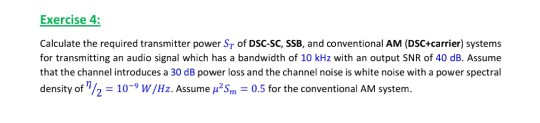comms systems theory: Exercise 4: Calculate the required transmitter power Sr of DSC-SC, SSB, and conventional AM (DSC+carrier) systems for transmitting an audio signal which has a bandwidth of 10 kHz with an output SNR of 40 dB. Assume that the channel introduces a 30 dB power loss and the channel noise is white noise with a power spectral density of η/2-10-9 W/Hz. Assume μ2Sm-0.5 for the conventional AM system. Exercise 4: Calculate the required transmitter power Sr of DSC-SC,...

• ### Station USAECE is at 950 on your AM dial and will broadcast from Shelby Hall. We...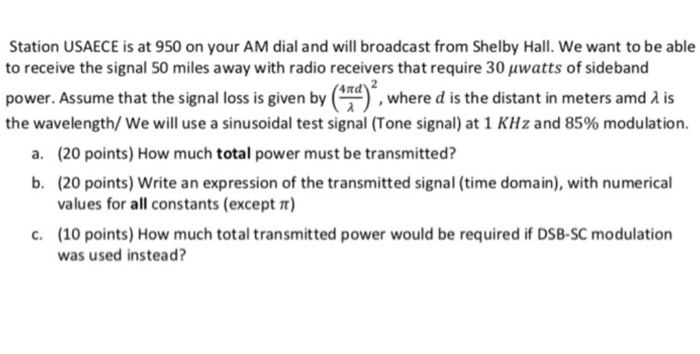Station USAECE is at 950 on your AM dial and will broadcast from Shelby Hall. We want to be able to receive the signal 50 miles away with radio receivers that require 30 uwatts of sideband power. Assume that the signal loss is given by the wavelength/ We will use a sinusoidal test signal (Tone signal) at 1 KHz and 85% modulation. where d is the distant in meters amd A is (20 points) How much total power must be...

• ### 1 2 4. The amplitude modulation signal is given by the following expression and is applied...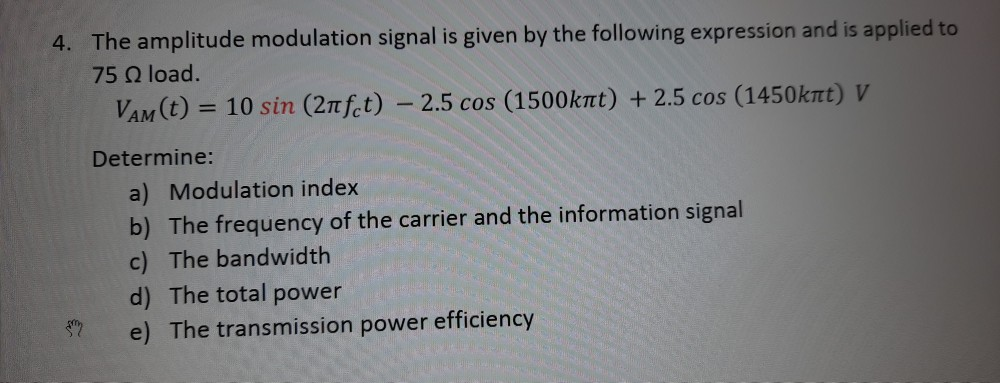1 2 4. The amplitude modulation signal is given by the following expression and is applied to 75 load. Vam(t) = 10 sin (21fct) - 2.5 cos (1500krt) + 2.5 cos (1450krt) V Determine: a) Modulation index b) The frequency of the carrier and the information signal c) The bandwidth d) The total power e) The transmission power efficiency be ar e 5. One of the inputs to a frequency modulator is a 250 kHz carrier signal with amplitude of...

• ### Question 1 0/1 pts An AM broadcast transmitter is modulated with: m (t)A cos 2Tfit + B cos 2Tf2t ...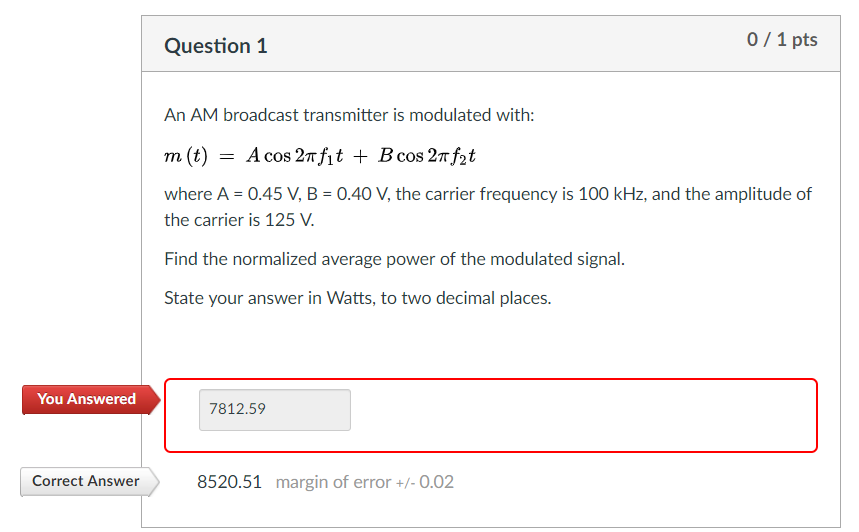Question 1 0/1 pts An AM broadcast transmitter is modulated with: m (t)A cos 2Tfit + B cos 2Tf2t where A 0.45 V B- 0.40 V, the carrier frequency is 100 kHz, and the amplitude of the carrier is 125 V. Find the normalized average power of the modulated signal State your answer in Watts, to two decimal places. You Answered 7812.59 Correct Answer 8520.51 margin of error+/-0.02 Question 1 0/1 pts An AM broadcast transmitter is modulated with: m...

• ### I am stuck with the problem of finding the average input power(dun know how to find...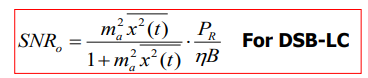I am stuck with the problem of finding the average input power(dun know how to find bar x(t)^2), with the formula below. Please help me and finish the question, and also in part b, the FM problem(how to find Pr). Thx. We were unable to transcribe this imageSNR3p SNR,-β2 for FM for FM Question 2 A communication channel has 20-dB attenuation and additive white Gaussian noise with power spectral density of 3x103 WHz. The bandwidth of the message signal is...

• ### Please show all the necessary steps to get the answers Answer Q5 A given DSB-LC transmitter...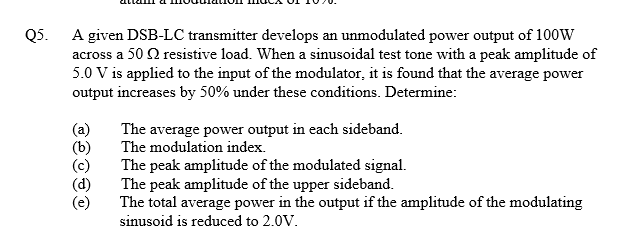Please show all the necessary steps to get the answers Answer Q5 A given DSB-LC transmitter develops an unmodulated power output of 100W across a 50 ? resistive load. When a sinusoidal test tone with a peak amplitude of 5.0 V is applied to the input of the modulator, it is found that the average power output increases by 50% under these conditions. Determine (a) (b) (c) (d) (e) The average power output in each sideband. The modulation index. The...